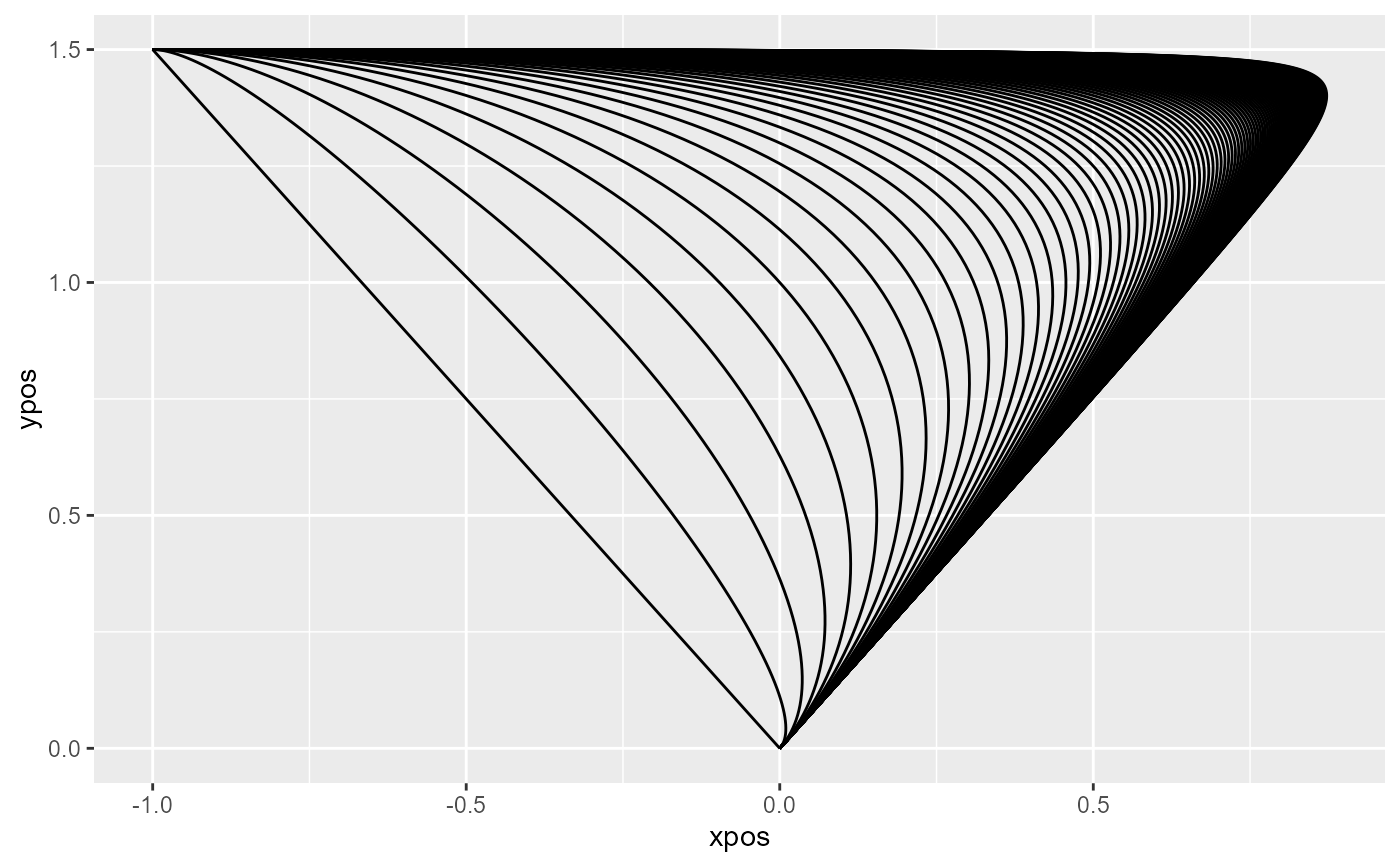bezier creates 3-point Bezier-curves using the Bernstein approximation to simulate continuous competition in mouse- and hand-trajectories.

bezier(x = c(0, 1, -1), y = c(0, 1.5, 1.5), w = 1, resol = 100)

## Arguments

x a numeric vector giving the x-coordinates of exactly three Bezier-points. Defaults to c(0,1,-1) matching the 'mt' format in mt_align. a numeric vector giving the x-coordinates of exactly three Bezier-points. Defaults to c(0,1.5,1.5) matching the 'mt' format in mt_align. a numeric value or vector specifying one or several Bezier curves, with w governing the pull towards the middle point. Each entry in w creates one Bezier-curve. a numeric value specifying the spatial resolution of the bezier curves. For example, resol = 100 creates bezier curves comprised of 100 points each.

## Value

A trajectory array containing the bezier curves.

## Examples

# Generate range of Bezier-curves
bezier_curves <- bezier(w=seq(0,10,.1))

# Plot curves
mt_plot(bezier_curves)